Subject:
Geometry
Material Type:
Lesson Plan
Level:
Middle School
7
Provider:
Pearson
Tags:
Language:
English
Media Formats:
Text/HTML

# Exploring The Properties Of A Triangle## Overview

Students explore properties of triangles. They fold paper to make a triangle and measure its angles. Students also draw triangles with given conditions.

# Key Concepts

The sum of the measures of the angles in a triangle is always 180°.

Given different side measures and/or angle measures, some scenarios will create triangles and others will not. Students explore various cases and draw conclusions about which conditions create triangles and why.

# Goals and Learning Objectives

• Draw triangles with given conditions.
• Find the sum of the measures of the angles of a triangle.
• Explore conditions that result in triangles.

# Lesson Guide

Have students view the opening figure and discuss the questions, “What do you know about his figure?” and “What makes a triangle a triangle?”

ELL: Encourage students to verbalize their explanations. Allow students to speak in small groups to gain confidence.

# Mathematics

Briefly allow students to share their observations without discussion. Students may notice:

• Triangles have three sides.
• Triangles have three angles.
• Triangles can have two, three, or no congruent sides.
• Triangles can have two, three, or no congruent angles.

# What Makes a Triangle a Triangle?

• What makes a triangle a triangle?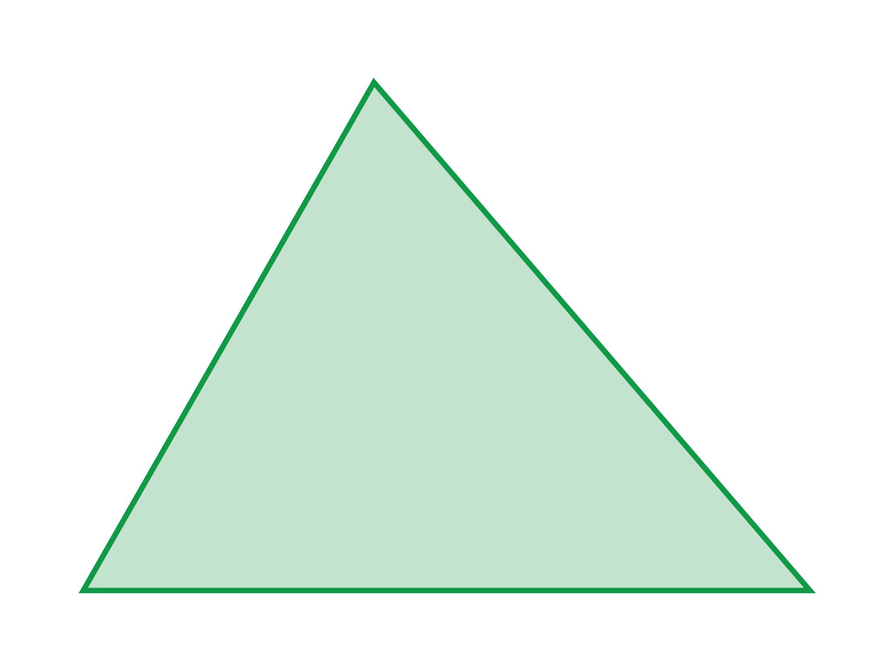# Lesson Guide

Discuss the Math Mission. Students will build triangles and explore the angle relationships in triangles.

## Opening

Build triangles and explore the angle relationships in triangles.

# Lesson Guide

Hand out protractors and 8½ in. x 11 in. sheets of paper. Tell students that compasses are available if they wish to use one. Have students work individually, but allow them to collaborate with a partner and share their results with their group or table.

SWD: If students seem unsure of how to start the task, model the steps for folding the paper, and model how to use the protractor with them before asking them to work on their task.

Have students who easily understand the task demonstrate the patterns they observed to struggling students. This promotes cooperative learning and is beneficial to both the stronger student and the student who is striving to learn the concept.

# Interventions

Student has difficulty getting started.

• Did your folds form a triangle? Why not?

The triangle shown is an example of what the folded sheet of paper might look like. It is labeled to make it easier to refer to specific angles.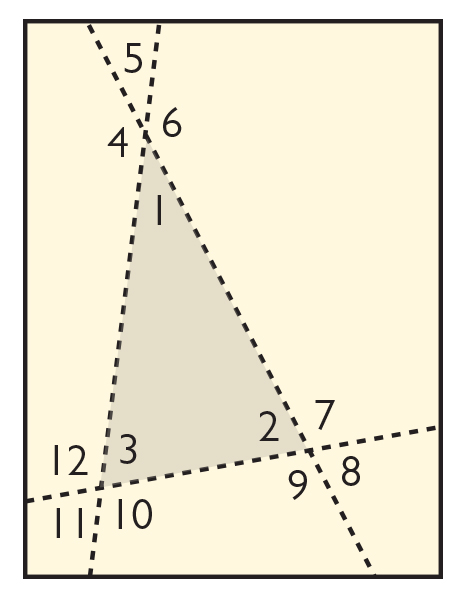• The figure is a triangle. It has three sides and three angles. A triangle was formed because each fold overlapped the other two, forming three intersections and three interior angles.
• Answers will vary. As they survey their neighbors, students see that the totals are not exactly 180°, but they should be close. Students should conclude that the measures of the angles add to 180°.
• Angles 1 and 5 and angles 4 and 6 are vertical angles and are congruent. Angles 1 and 6, angles 5 and 6, angles 4 and 5, and angles 1 and 4 are supplementary. With this knowledge, students can find the angle measures.

Note: Answers will vary. The numerical sum of the three angles will vary, but students should conclude that the sum of the three angles is equal to 360° minus the corresponding interior angle measure.

# Build Triangles

Start with a sheet of paper. Fold the left edge toward the center at a slant. Unfold. Fold the right edge toward the center so that the fold overlaps the first fold you made. Unfold. Fold the bottom edge toward the center so that the fold overlaps the first two folds you made. Unfold.

Look at the figure that is outlined by the folds.

• What type of figure is this? How do you know? Why do you think this figure was formed as a result of folding the paper the way you did?
• Measure each of the three angles inside the figure and write the measurements on the paper. What do you notice?
• For each of the three angles you measured, there are three angles that share the same vertex but lie outside the figure. What is the sum of these three angles? How do you know?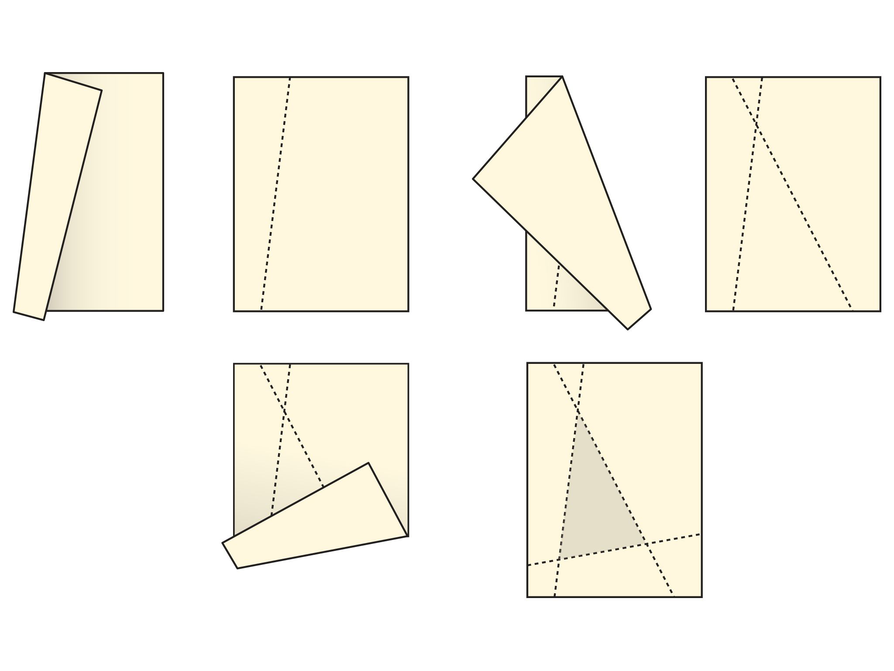## Hint:

• What is the sum of the angles in your triangle?
• Check with other students nearby. What is the angle sum in their triangles?

# Build Another Triangle

• The figure is a right triangle because the corner of the sheet is a right angle.
• One angle is a right angle and is 90°. The other two angles are complementary.

# Build Another Triangle

On the same sheet of paper, choose a corner and fold it toward the center. Unfold.

Look at the figure outlined by the edges of the paper and the fold.

• What type of figure is this? How do you know?
• Measure each of the angles. What do you notice?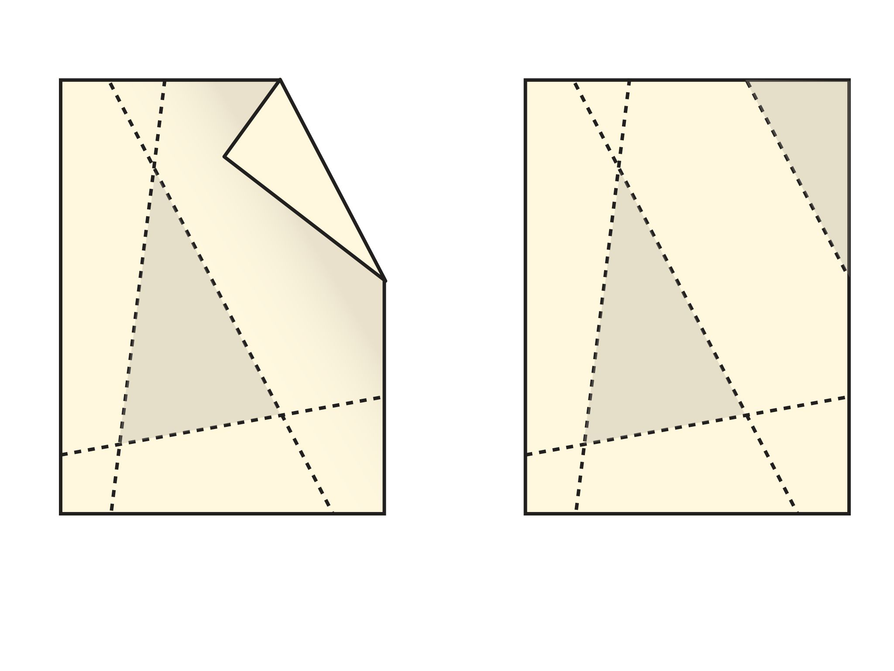# Interventions

Student has difficulty getting started.

• Is the measure of an obtuse angle greater than or less than 90°?
• What is the measure of each angle you are drawing?

Student has a solution.

• Why can't a triangle have two obtuse angles?
• What is true about the side lengths in order for them to form a triangle?

[common error] Student does not see that a triangle is a closed figure.

• How many angles does a triangle have?

• One angle measure could be (infinitely) close to 180°. The angle sum is still 180°, and the measures of the other two angles would be close to 0°.
• This triangle is not possible. The conclusion is that the sum of two angle measures in a triangle cannot be 180° or greater.
• Students will find it tricky to draw this triangle accurately. A compass will make the task easier. Teacher note: Students may need support when using a compass for making triangles with given side lengths. If students are struggling with using the appropriate tools, model how to use a compass in a small group.
• This triangle is not possible. Students should see that the sum of the lengths of two sides must be greater than the length of the third side.

# Draw Triangles

On a new sheet of paper, using your protractor and its ruler side, draw triangles with the following characteristics:

• Has one obtuse angle.
What is the largest angle measure possible for the obtuse angle? What can you conclude?
• Has two obtuse angles.
What do you notice? What can you conclude?
• Has side lengths of 3 inches, 4 inches, and
6 inches.
• Has side lengths of 3 inches, 4 inches, and
8 inches. What can you conclude?

# Preparing for Ways of Thinking

Look for these types of responses to share during the Ways of Thinking discussion:

• Students who found that the sum of the measures of the angles of a triangle is 180°.
• Students who realize that it is impossible to meet all the conditions for some problems.

# Mathematical Practices

Mathematical Practice 3: Construct viable arguments and critique the reasoning of others.

• Look for students who can make conjectures and generalize about properties of triangles based on the cases they have seen.

Mathematical Practice 5: Use appropriate tools strategically.

• Look for students who understand the limitations of the tools, particularly in drawing the triangle with given lengths, and ask for a compass.

Mathematical Practice 6: Attend to precision.

• Look for students who see the importance of measuring angles and lengths accurately and notice the effect on the final drawing. Students who do not attend to precision may not make the appropriate conclusions. Students understand that even though the angle sums of their triangle may not measure exactly 180°, the sum should be 180°.

Mathematical Practice 8: Look for and express regularity in repeated reasoning.

• Notice students who make the connection between supplementary angles and the sum of the angles in a triangle.

# Challenge Problem

• There are an infinite number of possible triangles. A compass makes the problem simple, as any radius (and angle) other than the two radii that are collinear with the third point (outside of the circle) could determine the third side.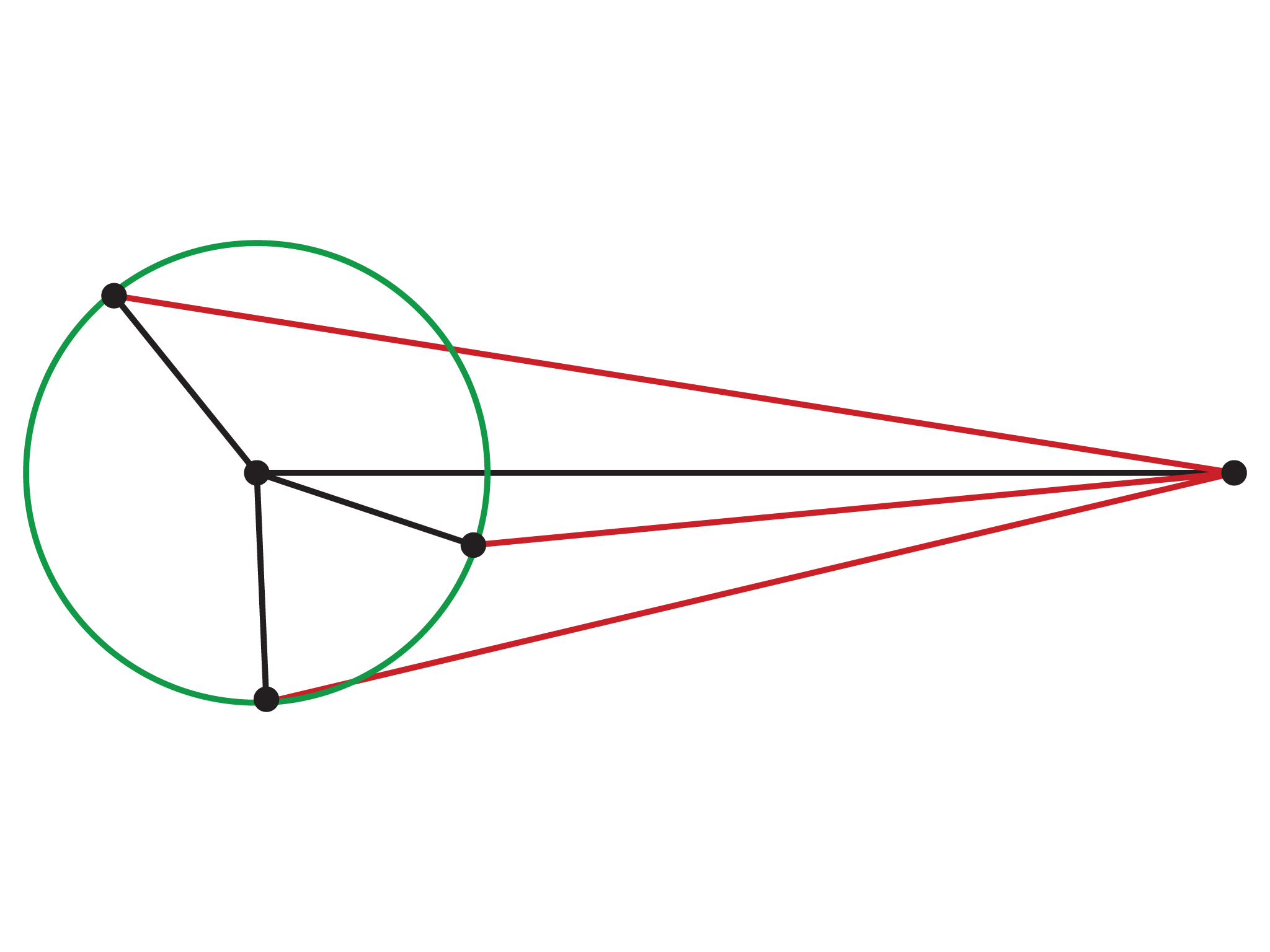# Prepare a Presentation

Explain how you constructed different types of triangles. Use your work to support your explanation.

# Challenge Problem

• Assume you were given two sides of a triangle. How many possible triangles can you draw?

# Ways of Thinking

Facilitate the discussion to help students understand the mathematics of the lesson. Ask questions such as the following:

• When you added the angle measures, was the sum exactly 180°? Why?
• What is the sum of the angle measures in a triangle?
• What is the greatest measure two angles in a triangle can have?
• Why is the measure of the outside supplementary angle (informally introduce the term exterior angle) the sum of the remaining two inside angles?
• How did you construct the triangles when you were given the lengths of the sides?
• How did [students] organize their thoughts differently? Which makes more sense to you? Which brought out the structure of the mathematics?
• How did [students] make sense of the problem?

ELL: Show these questions in writing and provide sentence frames such as the following to support ELLs:

• I found that the three angles of the triangle...
• The folded triangles showed...
• This makes sense to me because...

Post these sentence frames as a reference for future lessons.

# Ways of Thinking: Make Connections

## Hint:

• When you added up the angle measures, did the sum come to exactly 180°? Explain.
• What is the sum of the angle measures in a triangle?
• Why can’t a triangle have two obtuse angles?
• Within one triangle, what is the largest measure that the sum of angles can be?

# A Possible Summary

The sum of the angle measures in a triangle is 180°. The sum of any two angle measures in a triangle must be less than 180°, and the sum of the lengths of any two sides must be greater than the third side. There are certain conditions that will allow a triangle to be constructed and some that will not. For some conditions, more than one triangle can be made.

ELL: As with other discussions, encourage ELLs to use the academic vocabulary they have learned. Introduce new vocabulary as needed. As they participate in the discussion, be sure to monitor for knowledge of the topic.

# Summary of the Math: Triangles

Write a summary about the angles in a triangle.

## Hint:

• Do you say what the sum of the angle measures in a triangle is?
• Do you explain why it is impossible for a triangle to have two obtuse angles?
• Do you discuss what makes the shape of a triangle?